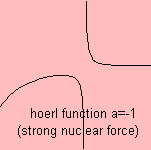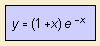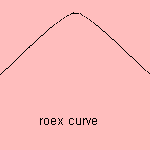# Hoerl function

## exponentional

last updated: 2005-01-02The Hoerl curve is used for curve fitting purposes.

strong nuclear force (a = -1)We find the curve in the formula for the strong nuclear force, use -x instead of x:The elementary particles of the atom's nucleus, are being held together by the strong nuclear force. The potential of this force, as function of the distance between the particles, is derived by the given formula.
In the strong nuclear force curve it's the part on the right side.

exponential curve (a=0)
For a=0 we find the exponential curve.a=1

A variation on the curve is used in the field of auditory filters, modeling the filter characteristics of the inner ear. The curve is the sum of a Hoerl function (a=1) and an exponential curve 1) :Adding a large number of such filters spaced across the audible frequency range, the rounded-exponential curve or roex curve, is formed (Patterson and Moore, 1986). This curve is slightly asymmetric, being wider on the low-frequency side.

The reciprocal of the Hoerl curve is found as the vapor pressure curve.

notes

1) y =  (1+x) e-x is equivalent to y = -x e-x + e-x.